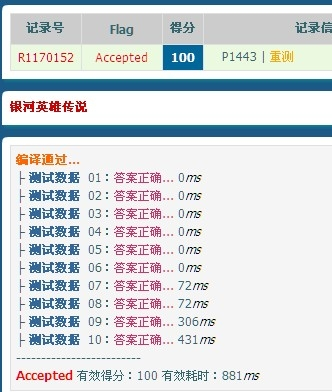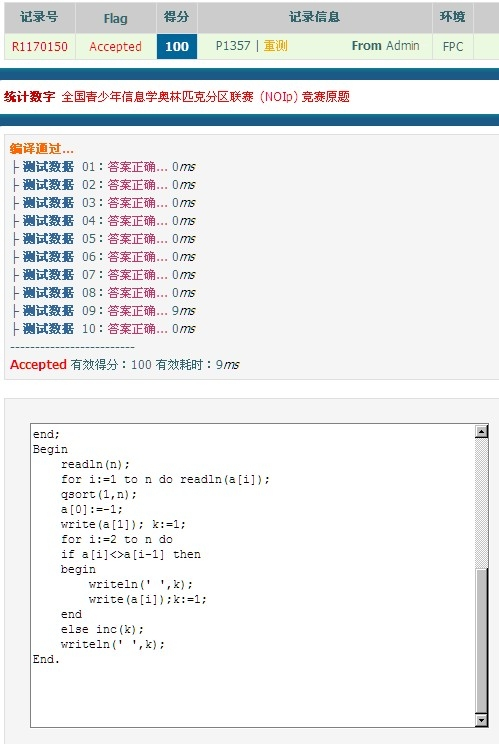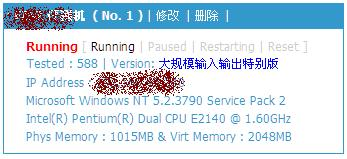Never Give Up！

New core？

## 解决Vijos大规模输入输出的BUG！## USACO/contact

{
ID:smdcnne1
PROG:contact
LANG:PASCAL
}
type _ans=record
x:longint;
s:string;
end;
var tot,i,j,k,l,m,a,b,n,g,last,p:longint;
st:ansistring; ch:char;
x:array[1..12]of longint;
f:array[1..12,0..10000]of longint;
t:array[1..12]of integer;
ans:array[0..50000]of _ans;
procedure qsort(l,r:longint);
var i,j:longint;x:_ans;
begin
i:=l;j:=r;x:=ans[(l+r)shr 1];
repeat
while (ans[i].x>x.x)or
((ans[i].x=x.x)and(length(ans[i].s)length(x.s)))or
((ans[j].x=x.x)and(length(ans[j].s)=length(x.s))and(ans[j].s>x.s))
do dec(j);
if i< =j then begin ans:=ans[i];ans[i]:=ans[j];ans[j]:=ans; inc(i);dec(j); end; until i>=j;
if il then qsort(l,j);
end;
begin
assign(input,'contact.in');
assign(output,'contact.out');
reset(input);rewrite(output);
st:='';
for i:=a to b do t[i]:=(1 shl (i-1))-1;
repeat
if ch in ['0'..'1'] then begin
inc(l);
for i:=a to b do
begin
x[i]:=x[i] and t[i];
x[i]:=x[i]*2+ord(ch)-ord('0');
if l>=i then inc(f[i,x[i]]);
end; end;
until eof;
tot:=0;
for i:=a to b do
begin
for j:=0 to (1 shl i)-1 do
begin
if f[i,j]>0 then
begin
inc(tot);
ans[tot].s:='';
ans[tot].x:=f[i,j];
g:=j;
for k:=1 to i do
begin
if (g and 1)>0 then ans[tot].s:='1'+ans[tot].s else ans[tot].s:='0'+ans[tot].s;
g:=g shr 1;
end;
end;
end;
end;
qsort(1,tot);
i:=1;
repeat
if ans[i].x<>last then
begin
dec(n);
if n>=0 then begin if i>1 then writeln; writeln(ans[i].x);
write(ans[i].s);
last:=ans[i].x; p:=1; end;
end else begin
inc(p);
if p mod 6=1 then begin writeln;write(ans[i].s)end else
write(' ',ans[i].s);
end;
inc(i);
until (n<0)or(i>tot);
writeln;
close(input);close(output);
end.

## 传说中的RP

———-华丽的分割线——-点击下载此文件